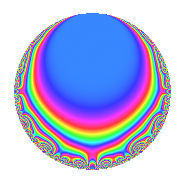# Properties

 Label 28.2.fLevel 28 Weight 2 Character orbit f Rep. character $$\chi_{28}(3,\cdot)$$ Character field $$\Q(\zeta_{6})$$ Dimension 4 Newforms 1 Sturm bound 8 Trace bound 0

# Related objects

## Defining parameters

 Level: $$N$$ = $$28 = 2^{2} \cdot 7$$ Weight: $$k$$ = $$2$$ Character orbit: $$[\chi]$$ = 28.f (of order $$6$$ and degree $$2$$) Character conductor: $$\operatorname{cond}(\chi)$$ = $$28$$ Character field: $$\Q(\zeta_{6})$$ Newforms: $$1$$ Sturm bound: $$8$$ Trace bound: $$0$$

## Dimensions

The following table gives the dimensions of various subspaces of $$M_{2}(28, [\chi])$$.

Total New Old
Modular forms 12 12 0
Cusp forms 4 4 0
Eisenstein series 8 8 0

## Trace form

 $$4q$$ $$\mathstrut -\mathstrut 2q^{2}$$ $$\mathstrut -\mathstrut 6q^{5}$$ $$\mathstrut -\mathstrut 8q^{8}$$ $$\mathstrut +\mathstrut O(q^{10})$$ $$4q$$ $$\mathstrut -\mathstrut 2q^{2}$$ $$\mathstrut -\mathstrut 6q^{5}$$ $$\mathstrut -\mathstrut 8q^{8}$$ $$\mathstrut +\mathstrut 6q^{10}$$ $$\mathstrut +\mathstrut 12q^{12}$$ $$\mathstrut +\mathstrut 2q^{14}$$ $$\mathstrut +\mathstrut 8q^{16}$$ $$\mathstrut -\mathstrut 6q^{17}$$ $$\mathstrut +\mathstrut 6q^{21}$$ $$\mathstrut -\mathstrut 4q^{22}$$ $$\mathstrut -\mathstrut 12q^{24}$$ $$\mathstrut -\mathstrut 4q^{25}$$ $$\mathstrut -\mathstrut 12q^{26}$$ $$\mathstrut -\mathstrut 20q^{28}$$ $$\mathstrut +\mathstrut 16q^{29}$$ $$\mathstrut -\mathstrut 6q^{30}$$ $$\mathstrut +\mathstrut 8q^{32}$$ $$\mathstrut +\mathstrut 6q^{33}$$ $$\mathstrut -\mathstrut 6q^{37}$$ $$\mathstrut +\mathstrut 18q^{38}$$ $$\mathstrut +\mathstrut 12q^{40}$$ $$\mathstrut +\mathstrut 12q^{42}$$ $$\mathstrut +\mathstrut 4q^{44}$$ $$\mathstrut -\mathstrut 2q^{46}$$ $$\mathstrut -\mathstrut 4q^{49}$$ $$\mathstrut +\mathstrut 8q^{50}$$ $$\mathstrut +\mathstrut 2q^{53}$$ $$\mathstrut -\mathstrut 18q^{54}$$ $$\mathstrut +\mathstrut 16q^{56}$$ $$\mathstrut -\mathstrut 36q^{57}$$ $$\mathstrut -\mathstrut 8q^{58}$$ $$\mathstrut -\mathstrut 12q^{60}$$ $$\mathstrut -\mathstrut 18q^{61}$$ $$\mathstrut +\mathstrut 12q^{65}$$ $$\mathstrut -\mathstrut 6q^{66}$$ $$\mathstrut +\mathstrut 6q^{70}$$ $$\mathstrut +\mathstrut 30q^{73}$$ $$\mathstrut -\mathstrut 6q^{74}$$ $$\mathstrut -\mathstrut 10q^{77}$$ $$\mathstrut +\mathstrut 24q^{78}$$ $$\mathstrut -\mathstrut 24q^{80}$$ $$\mathstrut +\mathstrut 18q^{81}$$ $$\mathstrut +\mathstrut 12q^{82}$$ $$\mathstrut +\mathstrut 12q^{85}$$ $$\mathstrut -\mathstrut 4q^{86}$$ $$\mathstrut +\mathstrut 4q^{88}$$ $$\mathstrut +\mathstrut 54q^{89}$$ $$\mathstrut +\mathstrut 8q^{92}$$ $$\mathstrut +\mathstrut 6q^{93}$$ $$\mathstrut -\mathstrut 30q^{94}$$ $$\mathstrut -\mathstrut 24q^{96}$$ $$\mathstrut -\mathstrut 22q^{98}$$ $$\mathstrut +\mathstrut O(q^{100})$$

## Decomposition of $$S_{2}^{\mathrm{new}}(28, [\chi])$$ into irreducible Hecke orbits

Label Dim. $$A$$ Field CM Traces $q$-expansion
$$a_2$$ $$a_3$$ $$a_5$$ $$a_7$$
28.2.f.a $$4$$ $$0.224$$ $$\Q(\zeta_{12})$$ None $$-2$$ $$0$$ $$-6$$ $$0$$ $$q+(-\zeta_{12}-\zeta_{12}^{2}+\zeta_{12}^{3})q^{2}+(\zeta_{12}+\cdots)q^{3}+\cdots$$